# High School Chemistry : Types of Reactions

## Example Questions

← Previous 1

### Example Question #23 : Chemical Reactions

Which of the following reactions is an example of an addition reaction?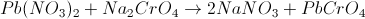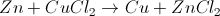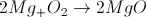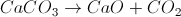Explanation: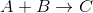When magnesium combines with oxygen gas, it results in a compound with both of them in it. This makes it a simple addition reaction. In general, addition reactions have a greater number of reactant compounds than product compounds.

The other answer choices correspond to the reactions below:

Decomposition reaction:Single-replacement reaction:Double-replacement reaction:### Example Question #24 : Chemical Reactions

What is another term for an addition reaction?

Single-replacement reaction

Double-replacement reaction

Hydrolysis reaction

Synthesis reaction

Decomposition reaction

Synthesis reaction

Explanation:

Addition reactions occur when two molecules combine to form a larger one, leading to a release of energy. Addition reactions result in the synthesis of a new molecule, giving them their second name: synthesis reactions.Many synthesis reactions can also be classified as dehydration reactions, if water is formed as a byproduct. One example of this is the addition of amino acids to form protein chains. Hydrolysis reactions use water as a reactant and are used to split molecules apart (decomposition).

### Example Question #1 : Help With Dissociation Reactions

What is another term for a dissociation reaction?

Dehydration reaction

Single-replacement reaction

Double-replacement reaction

Decomposition reaction

Synthesis reaction

Decomposition reaction

Explanation:

Dissociation reactions occur when one molecule is divided to form two smaller ones, leading to a decrease in energy. Dissociation reactions result in the break down of a large molecule to form smaller products, giving them their second name: decomposition reactions.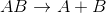Many decomposition reactions can also be classified as hydrolysis reactions, if water is used as a reactant. One example of this is the breakdown of glycogen to form glucose monomers. Dehydration reactions release water in order to form a new bond, building a larger molecule from smaller reactants (synthesis).

### Example Question #1 : Introduction For Reactions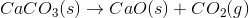The reaction above could be classified as __________.

decomposition

decomposition and oxidation-reduction

oxidation-reduction

synthesis

single displacement

decomposition

Explanation:

When one molecule is converted into two or more smaller molecules, the reaction is considered a decomposition reaction. In this example, none of the elements under go a change in oxidation number, so this is not an oxidation-reduction reaction. (Calcium is always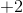, oxygen is always, and carbon remains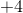throughout the reaction.)

### Example Question #3 : Help With Dissociation Reactions

In a dissociation reaction, determine the potential products for: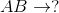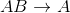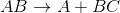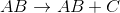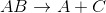Explanation:

Dissociation reactions usually entail a salt or ionic compounds dissociating or splitting into its components. In this case,where AB is made up of the components A and B. This reaction is ideally reversible even though the question does not provide the reversible arrows.

The options that include a third species, would be incorrect, as there are only two species in the original compound that is dissociating.

### Example Question #2 : Electrochemistry

Which of the following reactions involves both reduction and oxidation reactions?

Single displacement reactions

The reaction of sodium chloride and calcium sulfate

Neither single displacement reactions nor the reaction of sodium chloride and calcium sulfate

Both single displacement reactions and the reaction of sodium chloride and calcium sulfate

Single displacement reactions

Explanation:

A reaction that has both reduction and oxidation half reaction is called a redox reaction. It involves one or more atoms gaining electrons (reduction) and one or more atoms losing electrons (oxidation). Recall that single displacement reactions involve the replacement of an element in a compound with another element. An example of single replacement reaction is shown below: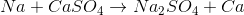In this reaction, a sodium atom replaces a calcium atom in calcium sulfate. If we calculate the oxidation numbers for sodium and calcium, we can see that sodium loses an electron (the oxidation state goes fromto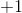) whereas the calcium ion gains two electrons (goes fromto); therefore, this is a redox reaction. All single displacement reactions follow this general trend and are characterized as redox reactions.

Reaction of sodium chloride and calcium sulfate is as follows: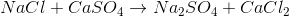If we calculate the oxidation state of each atom we will notice that oxidation number doesn’t change; therefore, this isn’t a redox reaction.

### Example Question #1 : Types Of Reactions

What is the type of reaction seen below?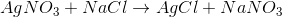Single-replacement

Double-replacement

Decomposition

Double-replacement

Explanation:

By looking at the reaction, we see that the cations for the reactants have their anions switched in the products. This means that the reaction follows the general outline: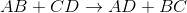This is an example of a double-replacement reaction.

Addition reactions convert two reactants to a single product, while decomposition reactions convert a single reactant to multiple products. Single-replacement reactions only switch one cation-anion pair.

### Example Question #2 : Help With Double Replacement Reactions

Predict the products for the following reaction if it were double displacement.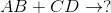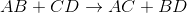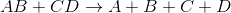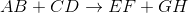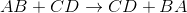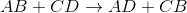Explanation:

In a double displacement reaction, or double replacement reaction, opposite ions combine. What this means is that the cation of one compound will recombine and bond to the anion of the other compound and vice versa.

In this example, we have to remember the ideal convention of cations being written before the anion in the compound. So in AB, we can presume A being the cation and B being the anion. The same goes for CD - C is the cation and D is the anion.

With this in mind, we can now easily see how the replacement would be possible.

If A is a cation, and was originally bound to B (anion), the only other anion it is left with to bind is D. If C is a cation, and was originally bound to D (anion), it is only left with the option of binding to the now-free B anion.

That's why it is:### Example Question #1 : Help With Oxidation Reduction Reactions

What process could be used to describe the conversion of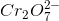to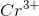?

Oxidation

Reduction

Combustion

Neutralization

Reduction

Explanation:

The process illustrated is the reduction of the chromium atom. Reduction occurs when electrons are gained, causing the oxidation number (charge) of the atom to decrease. We can examine this process by looking at the oxidations numbers of each atom involved.Oxygen always has an oxidation number of, and the ion has a total charge of. This allows us to find the oxidation number for chromium. Note that there are two chromium atoms and seven oxygen atoms in the ion.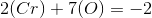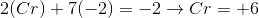The initial oxidation state of chromium is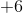.Following the reaction, chromium has a charge of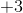, meaning that it also has an oxidation number of.

During the process, the chromium atom went from an oxidation state ofto an oxidation state of. This means that it must have gained electrons (reducing the oxidation state). This is a reduction process.

### Example Question #2 : Help With Oxidation Reduction Reactions

Determine which compound or ion is the oxidizing agent in the given reaction.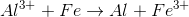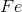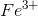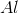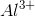Explanation:

The oxidizing agent is the element that causes the other element to be oxidized, and is itself being reduced in the equation. Reduction is defined as the gain of electrons, meaning the element would get "more negative."

In this reaction, aluminum transitions from an oxidation state of 3+ to an oxidation state of 0. This indicates a reduction, as the atom has gained more electrons and reduced charge.is thus the oxidizing agent in this reaction.

← Previous 1

### All High School Chemistry Resources